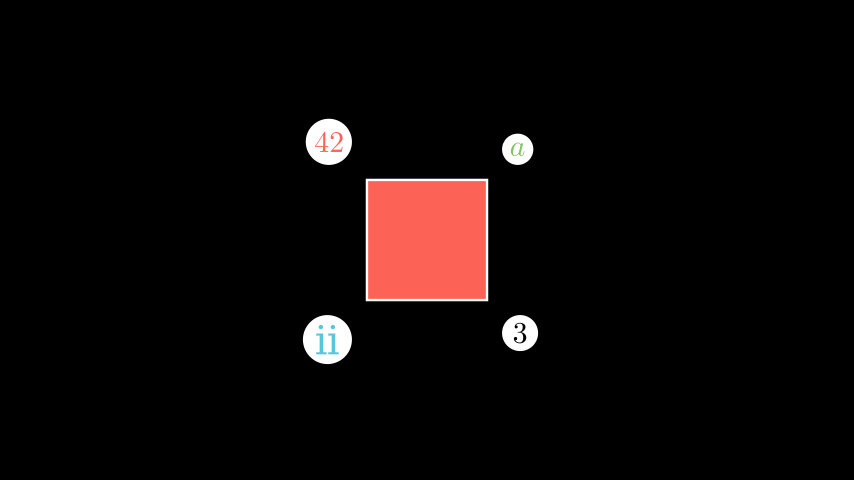# LabeledDot#

Qualified name: `manim.mobject.geometry.arc.LabeledDot`

Bases: `Dot`

A `Dot` containing a label in its center.

Parameters:

Examples

Example: SeveralLabeledDots```from manim import *

class SeveralLabeledDots(Scene):
def construct(self):
sq = Square(fill_color=RED, fill_opacity=1)
dot1 = LabeledDot(Tex("42", color=RED))
dot2 = LabeledDot(MathTex("a", color=GREEN))
dot3 = LabeledDot(Text("ii", color=BLUE))
dot4 = LabeledDot("3")
dot1.next_to(sq, UL)
dot2.next_to(sq, UR)
dot3.next_to(sq, DL)
dot4.next_to(sq, DR)
```
```class SeveralLabeledDots(Scene):
def construct(self):
sq = Square(fill_color=RED, fill_opacity=1)
dot1 = LabeledDot(Tex("42", color=RED))
dot2 = LabeledDot(MathTex("a", color=GREEN))
dot3 = LabeledDot(Text("ii", color=BLUE))
dot4 = LabeledDot("3")
dot1.next_to(sq, UL)
dot2.next_to(sq, UR)
dot3.next_to(sq, DL)
dot4.next_to(sq, DR)

```

Methods

Attributes

 `animate` Used to animate the application of any method of `self`. `animation_overrides` `color` `depth` The depth of the mobject. `fill_color` If there are multiple colors (for gradient) this returns the first one `height` The height of the mobject. `n_points_per_curve` `sheen_factor` `stroke_color` `width` The width of the mobject.